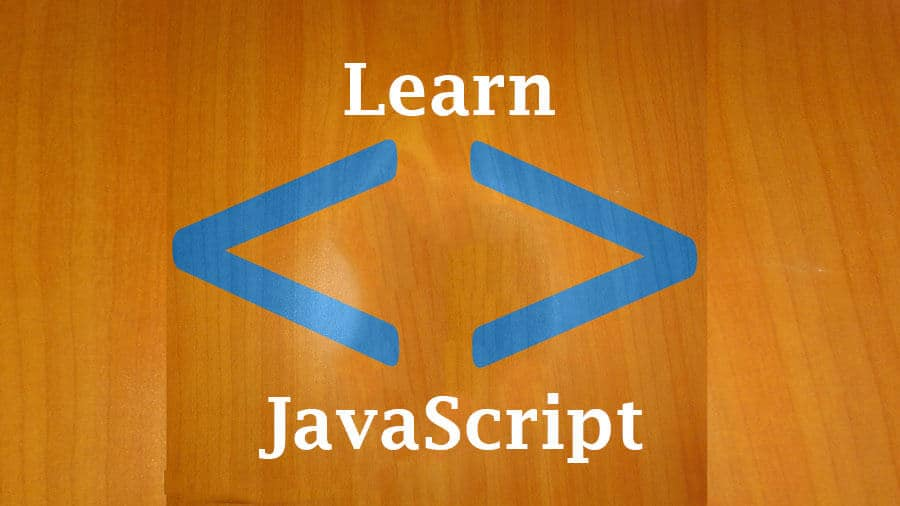# 33 JAVASCRIPT Literals – JSLast updated on June 30th, 2018 at 08:56 pm

This video is taken from the full course that will teach you HTML, CSS, Programming concepts and Javascript .

Transcript :

Hi, and welcome back.

So in this section, let’s start looking at literals.

Literals represent the actual values that you use in the code.

These actual values are the values that get computed.

For example, if you have a number lik 2, this is an actual value.

Maybe this statement says 2+2.

These are literals.

These are actual numbers.

Maybe you have something that gives out a statement like “Hi”.

This is a literal.

So literals are the actual values.

So we have different types of literals.

We have integer literals that refers to the numbers.

Maybe 2, it can be -4, -1 million, you know, different, different kinds of numbers.

Integer numbers, then we also have floating points.

Now floating points, these are numbers that have decimals.

So 2.11, that’s a floating point number.

It can also be in the form of an exponential.

So 2e-4, this is the same as 2/10000 which essentially is the same as 0.0002.

So, that’s a floating point.

It has a decimal and a number that follows the decimal or they follow the decimal, can even be zero.

This is also a floating point number and it can have an exponential or t can be a fraction.

So this part is the fraction.

Then we have strings.

Then we have strings.

A string literal, a string literal contains one or more characters.

So, in this one we said “Hi”, “Hi”, that’s a string literal.

“My name is somebody blah blah blah blah”, that’s a sting literal.

Then we have Booleans.

Booleans only have 2 possible values.

And these 2 possible values are either 2 or false.

Then we have arrays.

An array literal, this one has a group of elements, different elements, can be numbers.

In JavaScript, they can be a mixture of numbers.

And, let us, it can even be a statement.

In the, so that’s an array.

We’re going to look at each and every one of these, each of them.

Then we’ll also look at object literals and regular expressions.

So objects, objects are key value pairs.

So maybe you have an item there, key1, and then the value.

So we’re going to look at each and every one of these.

So that’s an object.

Then we have regular expressions.

So regular expressions, these are patterns used for pattern matching.

Maybe you have a statement that says “My name is X” and you want to match name, you want to make sure maybe name has a capital ‘N’.

So there’s a way you can do that using regular expressions and maybe you want to return back something.

Maybe return name.

So essentially, you can use regular expressions for patterns and you’re going to see how instrumental they’ll be in your career as a programmer.

They’re very instrumental.

And once you learn them, you’re going o like them.

So that’s it for this video.

I will see you in the next video when we get started looking at integer literals.

See you then.

Click the button below to watch this full course: